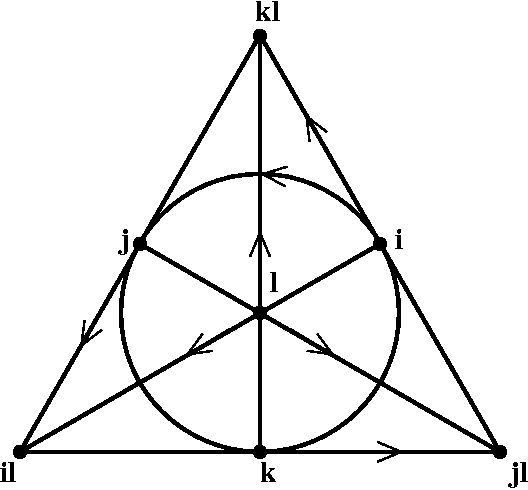## Octonions

The following brief introduction to the octonions is adapted from any of several papers
which can be found here, and which include further references to the literature.The octonions O are the nonassociative, noncommutative, normed division algebra over the real numbers. They can be expressed in terms of a natural basis, which we denote i,j,k,kl,jl,il,l, together with the identity element, 1. Each basis element except 1 squares to -1, that is i2=j2=-1, and so forth. The octonions are thus a generalization of the complex numbers, with 7 imaginary units rather than just one.

The full multiplication table is conveniently encoded in the 7-point projective plane, shown above. The product of any two imaginary units is given by the third unit on the unique line connecting them, with the sign determined by the relative orientation. For instance, i j=k, i l=il, as well as cyclic permutations of these relations, but j i=-k.

The associator of three octonions is [a,b,c] =(ab)c - a(bc) which is totally antisymmetric in its arguments and has no real part. Although the associator does not vanish in general, the octonions do satisfy a weak form of associativity known as alternativity, namely [a,b,a]=0. (The underlying reason for this is that two octonions determine a quaternionic subalgebra of the octonions, so that any product containing only two octonionic directions is associative.)

Octonionic conjugation is given by reversing the sign of the imaginary basis units. Conjugation is an antiautomorphism, since it satisfies (ab)* = b* a*. The inner product on O is the one inherited from R8, which can be rewritten a·b = (ab* + ba*)/2 = (b*a + a*b)/2. Finally, the norm of an octonion is just |a|2 = aa* which satisfies the defining property of a normed division algebra, namely |ab| = |a| |b|

tevian@math.orst.edu